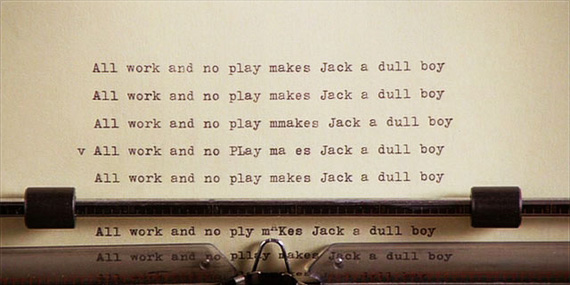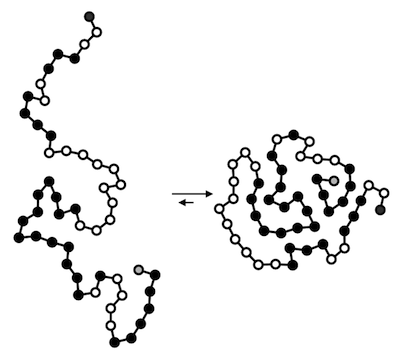Classical Mechanics

# Configurational entropy: Level 3-4 Challenges

A token is placed at one of 9 positions in a $$3 \times 3$$ grid according to a probability distribution $$P.$$ After a token is placed into one of the positions of the grid, it is then moved uniformly at random to one of the horizontally, vertically, or diagonally adjacent positions. For each position on the board, the probability that the token is in that position after being moved is also given by the distribution $$P.$$

If two tokens are placed into the grid according to the distribution $$P$$ and then moved, the probability that the set of occupied positions is the same before and after the tokens are moved can be expressed as $$\frac{a}{b}$$ where $$a$$ and $$b$$ are coprime positive integers. What is the value of $$a + b?$$A weight hangs at a steady height from a rubber band that is attached to the ceiling. Now, you turn on an air conditioner, significantly lowering the temperature of the room. What happens to the position of the weight?If you could read the title just fine, it is because the English language (as well as all natural languages) are redundant. This isn't to say that there are multiple words that mean the same thing (although there are), but that if you compare the number of questions you would have to ask to uniquely identify a word I'm thinking of, and the number of possible words, the second number is much bigger than the first.

For example, by the time you read the first four letters of a word starting with calc, you can be pretty sure it's going to end up being calculus, calcium, calculate, or calculation. You don't need all the extra letters to distinguish the remaining possibilities. Concretely, if we take a list of all the words of a given length that exist, and sort them into alphabetical order, we only need $$\log_2 N$$ questions to identify any given word in the list (where $$N$$ is the number of words in the list). On the other hand, if we were making full use of the language, we could manage $$\displaystyle 26^L$$ unique words of length $$L$$.

Using the English language dictionary built in to UNIX operating systems, and filtering for words of length 5, I find 10230 unique words. Taking words of length 5 as a proxy for the entire English language, how short, on average, could we make five letter words before someone with perfect reasoning couldn't read them anymore?

One thousand dust particles are trapped on a surface. The two states that a particle can occupy are

• absorbed on the surface in a zero energy state.
• excited with energy $$E_1$$.

Suppose the particles are in thermal equilibrium at temperature $$T$$, and 20 % of the particles are in the excited state. Find the value of $\frac{1}{k_BT}\sum_i E_i$

Details and Assumptions

• The particles are identical.
• $$k_B$$ is the Boltzmann constant.Proteins are molecules responsible for catalyzing chemical reactions, regulating gene expression, sensing changes in the extracellular environment, giving superstructure to the genome, and many other important tasks. They take the form of long chains that fold into compact structures by seeking out shapes that minimize the energy of self-interaction.

Consider a simple protein that has two states, completely folded, and completely unfolded, which have a free energy gap of $$\Delta = 3.74$$ kJ/mole.

You have an ensemble of several million copies of this protein dissolved in buffer. At temperature $$T = 37^{\circ}$$C, what fraction of the proteins are folded?